# NCERT Solution for class 8 maths chapter 5 - Data Handling

NCERT solutions for class 8 maths chapter-5 Data Handling is prepared by academic team of Physics Wallah. We have prepared NCERT solutions for all exercise of chapter-5. Given below is step by step solutions of all questions given in NCERT textbook for chapter-5. Read chapter-5 theory make sure you have gone through the theory part of chapter-5 from NCERT textbook and you have learned the formula of the given chapter. Physics Wallah prepared a detail notes and additional questions for class 8 maths with short notes of all maths formula of class 8 maths 5. Do read these contents before moving to solve the exercise of NCERT chapter-5.

### NCERT Solutions for Class 8 Maths Exercise 5.1

Question 1.

For which of these would you use a histogram to show the data:
(a) The number of letters for different areas in a postman’s bag.
(b) The height of competitors in an athletics meet.
(c) The number cassettes produced by 5 companies.
(d) The number of passengers boarding trains from 7.00 a.m. to 7.00 p.m. at a station.
Give reason for each.

Solution :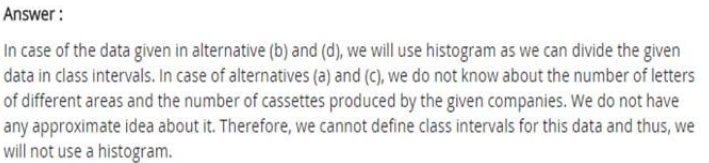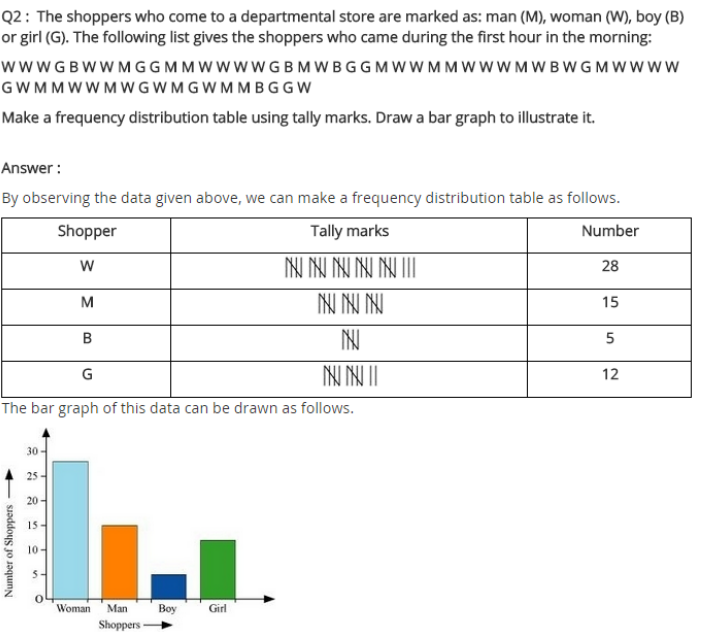Question 3.

The weekly wages (in `) of 30 workers in a factory are:
830, 835, 890, 810, 835, 836, 869, 845, 898, 890, 820, 860, 832, 833, 855, 845, 804, 808, 812, 840, 885, 835, 835, 836, 878, 840, 868, 890, 806, 840.
Using tally marks, make a frequency table with intervals as 800 – 810, 810 – 820 and so on.

Solution :
The representation of data by frequency distribution table using tally marks is as follows: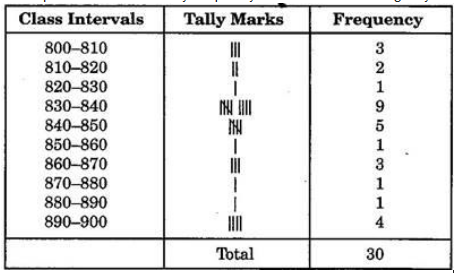Question 4.
Draw a histogram for the frequency table made for the data in Question 3 and answer the following questions.
(i) How many workers earn; 850 and more?
(ii) How many workers earn less than; 850?

Solution :
830 – 840 group has the maximum number of workers.
(i) 10 workers can earn more than; 850.
(ii) 20 workers earn less than; 850.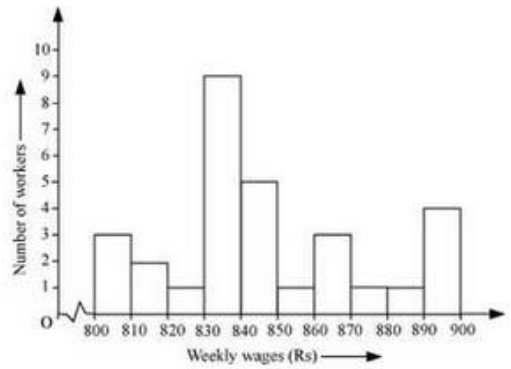Question 5.
The number of hours for which students of a particular class watched television during holidays is shown through the given graph.
We draw the histogram for above frequency table: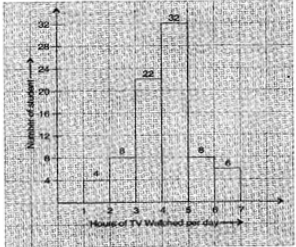(i) For how many hours did the maximum number of students watch T.V.?
(ii) How many students watched TV for less than 4 hours?
(iii) How many students spent more than 5 hours in watching TV?

Solution :
(i) The maximum number of students watched T.V. for 4 – 5 hours.
(ii) 34 students watched T.V. for less than 4 hours.
(iii) 14 students spent more than 5 hours in watching T.V.

### NCERT Solutions for Class 8 Maths Exercise 5.2

Question 1.
A survey was made to find the type of music that a certain group of young people liked in a city.
Adjoining pie chart shows the findings of this survey.
From this pie chart, answer the following:
(i) If 20 people liked classical music, how many young people were surveyed?
(ii) Which type of music is liked by the maximum number of people?
(iii) If a cassette company were to make 1000 CD’s, how many of each type would they make?

Solution :
(i) 10% represents 100 people.
Therefore 20% represents =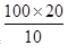= 200 people
Hence, 200 people were surveyed.
(ii) Light music is liked by the maximum number of people.
(iii) CD’s of classical music =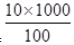= 100
CD’s of semi-classical music =;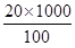= 200
CD’s of light music =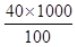= 400
CD’s of folk music =;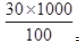= 300

Question 2.
A group of 360 people were asked to vote for their favourite season from the three seasons rainy, winter and summer.
(i) Which season got the most votes?
(ii) Find the central angle of each sector.
(iii) Draw a pie chart to show this information.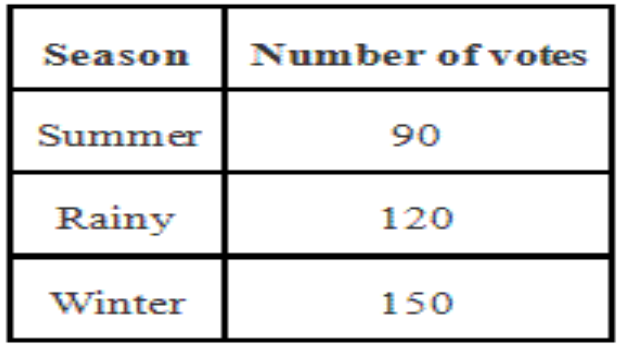Solution :

(i) Winter season got the most votes.
(ii) Central angle of summer season =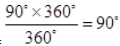Central angle of rainy season
=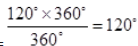Central angle of winter season
=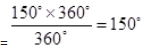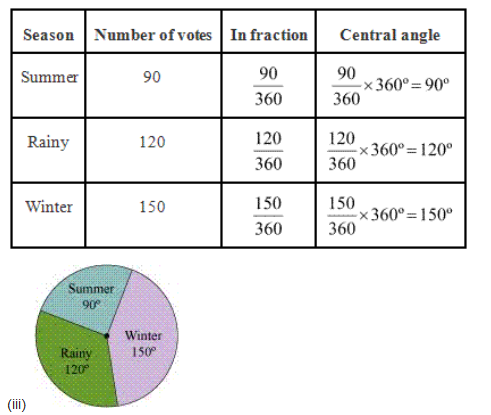Question 3.
Draw a pie chart showing the following information. The table shows the colours preferred by a group of people.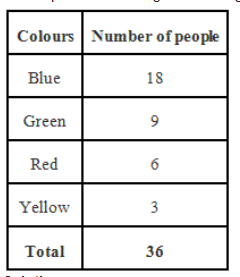Solution :
Here, central angle =; and total number of people = 36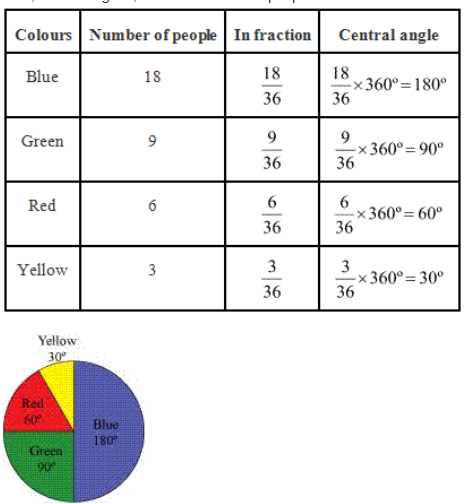Question 4.

The adjoining pie chart gives the marks scored in an examination by a student in Hindi, English, Mathematics, Social Science and Science. If the total marks obtained by the students were 540, answer the following questions: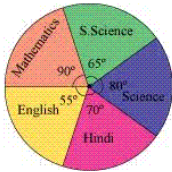(i) In which subject did the student score 105 marks?
(Hint: for 540 marks, the central angle 360º= So, for 105 marks, what is the central angle?)
(ii) How many more marks were obtained by the student in Mathematics than in Hindi?
(iii) Examine whether the sum of the marks obtained in Social Science and Mathematics is more than that in Science and Hindi.
(Hint: Just study the central angles)

Solution :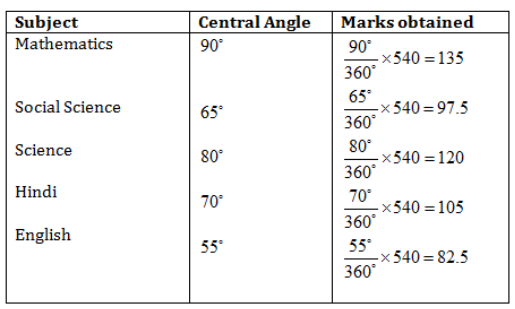(i) The student scored 105 marks in Hindi.
(ii) Marks obtained in Mathematics = 135
Marks obtained in Hindi = 105
Difference = 135 – 105 = 30
Thus, 30 more marks were obtained by the student in Mathematics than in Hindi.
(iii) The sum of marks in Social Science and Mathematics = 97.5 + 135 = 232.5
The sum of marks in Science and Hindi = 120 + 105 = 225
Yes, the sum of the marks in Social Science and Mathematics is more than that in Science and Hindi.

Question 5.
The number of students in a hostel, speaking different languages is given below.
Display the data in a pie chart.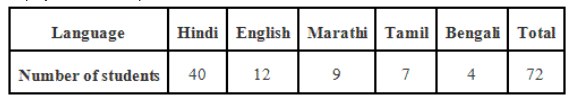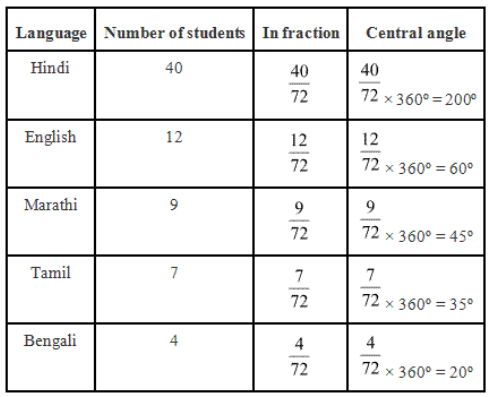Pie chart at above given data is as follow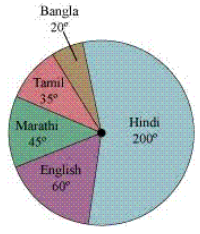### NCERT Solutions for Class 8 Maths Exercise 5.3

Question 1.
List the outcomes you can see in these experiments.
(a) Spinning a wheel ;
(b) Tossing two coins together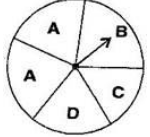Solution :
(a) There are four letters A, B, C and D in a spinning wheel. So there are 4 outcomes.
(b) When two coins are tossed together. There are four possible outcomes HH, HT, TH, TT.
(Here HT means head on first coin and tail on second coin and so on.)

Question 2.
When a die is thrown, list the outcomes of an event of getting:
(i) (a) a prime number; ;
(b) not a prime number
(ii) (a) a number greater than 5 ;
(b) a number not greater than 5

Solution :
(i) (a) Outcomes of event of getting a prime number are 2, 3 and 5.
(b) Outcomes of event of not getting a prime number are 1, 4 and 6.
(ii) (a) Outcomes of event of getting a number greater than 5 is 6.
(b) Outcomes of event of not getting a number greater than 5 are 1, 2, 3, 4 and 5.

Question 3.
Find the: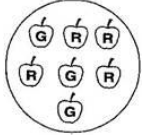(a) Probability of the pointer stopping on D in (Question 1 (a)).
(b) Probability of getting an ace from a well shuffled deck of 52 playing cards.
(c) Probability of getting a red apple. (See figure alongside)

Solution :

(a) In a spinning wheel, there are five pointers A, A, B, C, D. So there
are five outcomes. Pointer stops at D which is one outcome.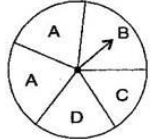So the probability of the pointer stopping on D =1/5
(b) There are 4 aces in a deck of 52 playing cards. So, there are four events of getting an ace.
So, probability of getting an ace =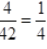(c) Total number of apples = 7
Number of red apples = 4
Probability of getting red apple =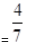Question 4.

Numbers 1 to 10 are written on ten separate slips (one number on one slip), kept in a box and mixed well. One slip is chosen from the box without looking into it. What is the probability of:
(i) getting a number 6.
(ii) getting a number less than 6.
(iii) getting a number greater than 6.
(iv) getting a 1-digit number.

Solution :
(i) Outcome of getting a number 6 from ten separate slips is one.
Therefore, probability of getting a number 6 =1/10
(ii) Numbers less than 6 are 1, 2, 3, 4 and 5 which are five. So there are 5 outcomes.
Therefore, probability of getting a number less than 6 =5/10 =1/2
(iii) Number greater than 6 out of ten that are 7, 8, 9, 10.So there are 4 possible outcomes.
Therefore, probability of getting a number greater than 6 = 4/10 = 2/5
(iv) One digit numbers are 1, 2, 3, 4, 5, 6, 7, 8, 9 out of ten.
Therefore, probability of getting a 1-digit number = 9/10

Question 5.
If you have a spinning wheel with 3 green sectors, 1 blue sector and 1 red sector, what is the probability of getting a green sector? What is the probability of getting a none-blue sector?

Solution :
There are five sectors. Three sectors are green out of five sectors.
Therefore, probability of getting a green sector = 3/5
There is one blue sector out of five sectors.
Non-blue sectors = 5 – 1 = 4 sectors
Therefore, probability of getting a non-blue sector = 4/5

Question 6.
Find the probability of the events given in Question 2.

Solution :
When a die is thrown, there are total six outcomes, i.e., 1, 2, 3, 4, 5 and 6.
(i) (a) 2, 3, 5 are prime numbers. So there are 3 outcomes out of 6.
Therefore, probability of getting a prime number =  3/6 = 1/2
(b) 1, 4, 6 are not the prime numbers. So there are 3 outcomes out of 6.
Therefore, probability of getting a prime number =3/6 = 1/2
;
(ii) (a) Only 6 is greater than 5. So there is one outcome out of 6.
Therefore, probability of getting a number greater than 5 =1/6
(b) Numbers not greater than 5 are 1, 2, 3, 4 and 5. So there are 5 outcomes out of 6.
Therefore, probability of not getting a number greater than 5 =5/6

### Notes,worksheet and solved question for Maths class 8

Check your marks in a chapter which you have complited in school from Physics Wallah chapter wise online test just click on the link given below